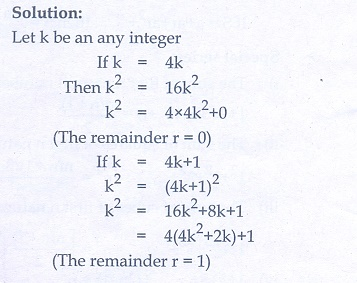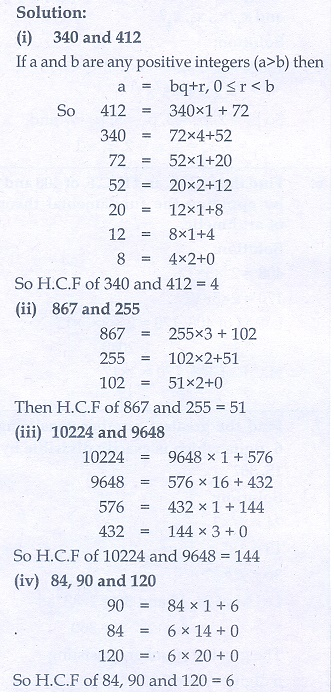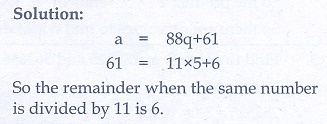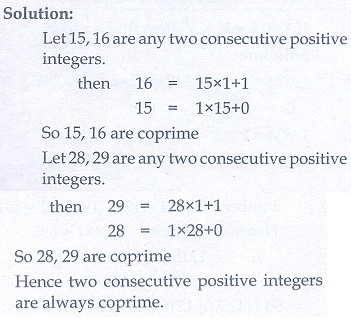Home | | Maths 10th Std | Exercise 2.1: Euclid’s Division Algorithm

# Exercise 2.1: Euclid’s Division Algorithm

Maths Book back answers and solution for Exercise questions - Mathematics : Numbers and Sequences: Euclid’s Division Algorithm: Exercise Problem Questions with Answer

Exercise 2.1

1. Find all positive integers, when divided by 3 leaves remainder 2.2. A man has 532 flower pots. He wants to arrange them in rows such that each row contains 21 flower pots. Find the number of completed rows and how many flower pots are left over.3. Prove that the product of two consecutive positive integers is divisible by 2.4. When the positive integers and are divided by 13, the respective remainders are9,7 and 10. Show that a+b+is divisible by 135. Prove that square of any integer leaves the remainder either 0 or 1 when divided by 4.6. Use Euclid’s Division Algorithm to find the Highest Common Factor (HCF) of

(i) 340 and 412

(ii) 867 and 255

(iii) 10224 and 9648

(iv) 84, 90 and 1207. Find the largest number which divides 1230 and 1926 leaving remainder 12 in each case.8. If is the Highest Common Factor of 32 and 60, find and satisfying = 32+ 60 .9. A positive integer when divided by 88 gives the remainder 61. What will be the remainder when the same number is divided by 11?10. Prove that two consecutive positive integers are always coprime.1. 2, 5, 8, 11, …

2. 25, 7

6.(i) 4 (ii) 51 (iii) 144 (iv) 6

7. 174

8. 2 ,-1

9. 6

Tags : Problem Questions with Answer, Solution | Mathematics , 10th Mathematics : UNIT 2 : Numbers and Sequences
Study Material, Lecturing Notes, Assignment, Reference, Wiki description explanation, brief detail
10th Mathematics : UNIT 2 : Numbers and Sequences : Exercise 2.1: Euclid’s Division Algorithm | Problem Questions with Answer, Solution | Mathematics